# GCSE Calculator Questions 1 (2013/Foundation Tier)

This worksheet contains a sample of practice questions based on the 2013 GCSE Foundation Level syllabus. Calculators may be used.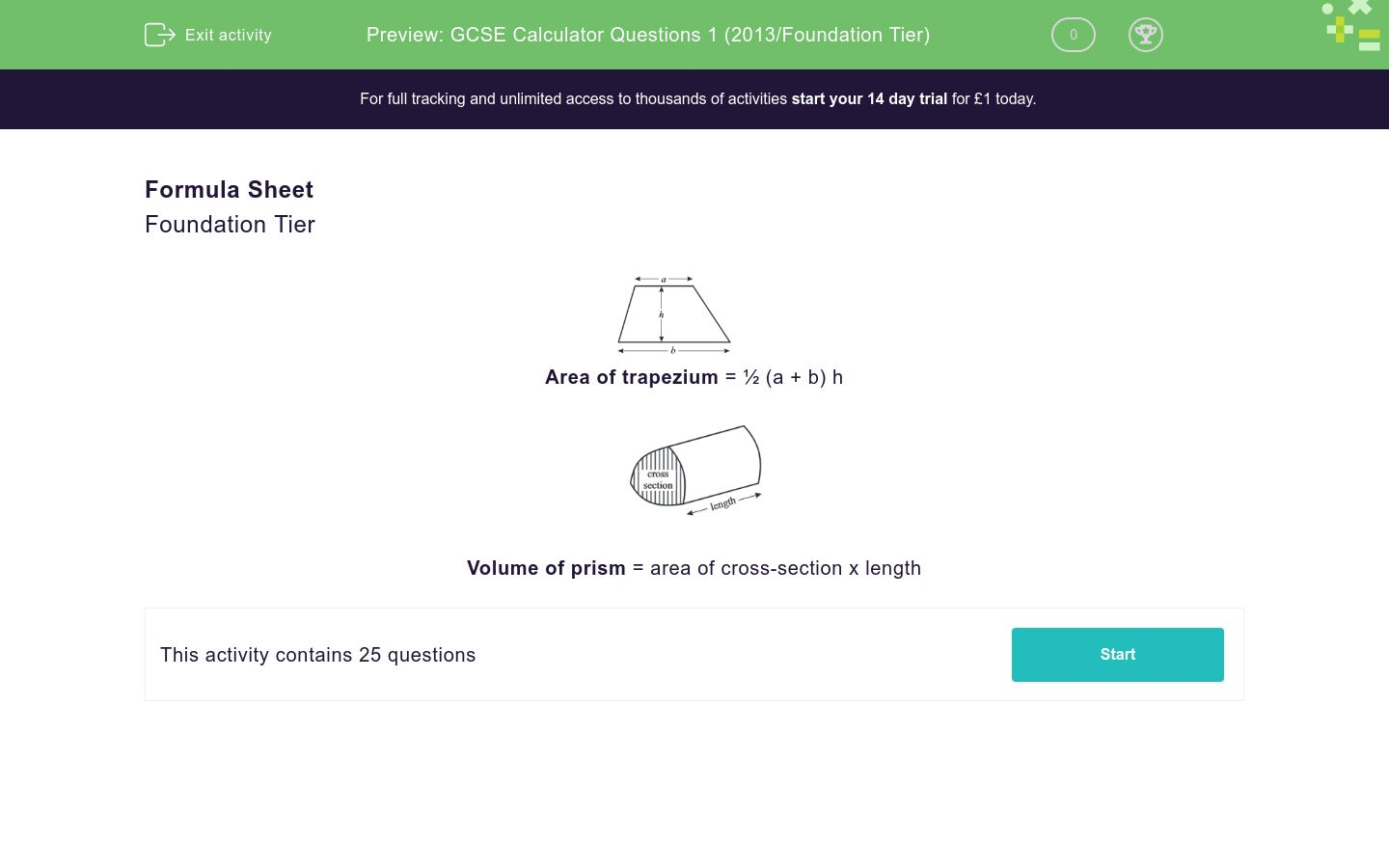Key stage:  KS 4

Curriculum topic:  GCSE Practice Papers

Curriculum subtopic:  Selection of Topics for Calculator Practice

Difficulty level:### QUESTION 1 of 10

Formula Sheet

Foundation TierArea of trapezium = ½ (a + b) hVolume of prism = area of cross-section x length

Last year, the following number of people went to visit Leonardo Da Vinci's Last Supper:

Three hundred and two thousand, five hundred and thirty-seven.

(Write this number in figures)

The diagram shows a protractor correctly positioned to measure the angle shown.

What is the size of the acute angle shown below.64°

76°

116°

124°

180°

Unlikely

Impossible

Certain

Likely

Even Chance

Match the words above to describe the likelihood of these five situations happening.

## Column B

There will be 100 days in December.
Certain
It will snow in Italy next August.
Unlikely
The next baby born in England will be a girl.
Even Chance
I will get at least one head if I throw a coin 100...
Likely
The sun will set tonight.
Impossible

Use a calculator to work out

the square root of 7.29

Work out:

¾ of 128

Pens cost £1.35 each.

Ann has £20 to spend on pens.  She buys the greatest number possible.

Work out the number of pens Ann buys.

Solve:

2y - 5 = 12

y = 2/7

y = 3½

y = 8

y = 8½

Calculate the area of the trapezium shown below in cm2.

(Just write the number)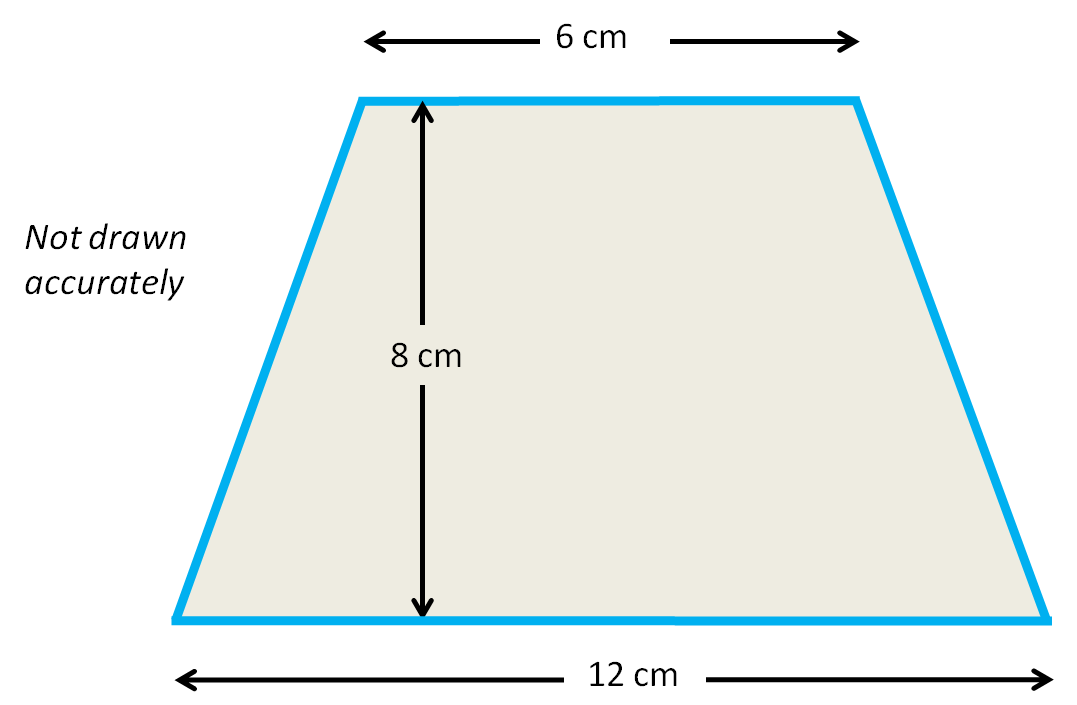Write down the value of

142

Write down the value of

43

From this list of number, match the answers.

15   17   19   21   23   25

## Column B

the smallest prime number
17
The largest prime number
25
A square number
21
A multiple of 7
23

Round this number to 2 significant figures:

18742

18.9 x 2.331

Ahmed goes on holiday to Italy and changes £750 into euros (€) before he goes.

The exchange rate is £1 = €1.12.

In Italy, he spends €480 euros and brings the remaining euros home.

He changes these euros back into pounds.

The exchange rate is now £1 = €1.20.

How much money in pounds does he get back?

(Just write the number)

Simplify

5a + 8b - 2a + b

Calculate the area of the following shape made of rectangular pieces.

(Just write the number)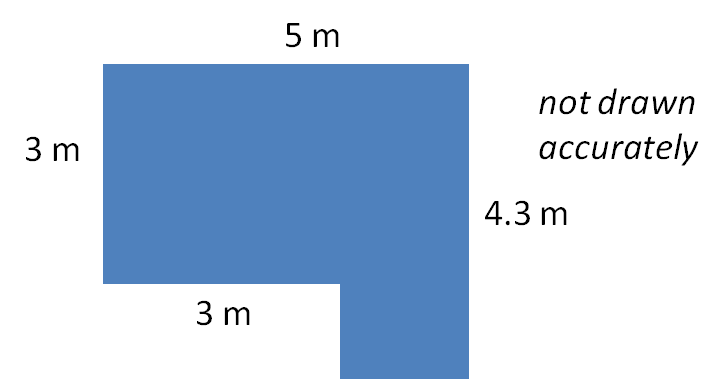The Triangle ABC is isosceles.

Calculate the angle marked a°.Calculate the length of the side BC in cm in the following triangle.

(Just write the number to 2 decimal places)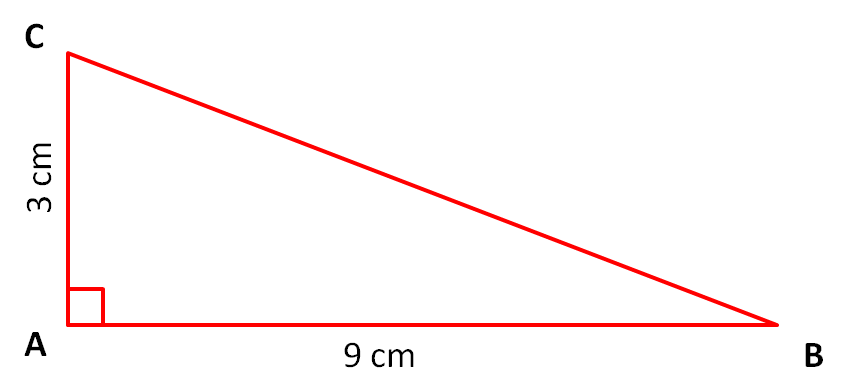Here is a sign showing the prices for using a car park.

 CAR PARK £1.75 for the first hour £1.25 for each hour after

Jane pays £9.25 to park.

For how many hours does she park?

(Just write the number)

Match the most suitable measurement to each item.

## Column B

Mass of a drawing pin
56cm
Mass of an apple
56m
Height of a bus
100g
Height of a table
4.4m
Height of Tower of Pisa
1g

Use trial and improvement to find a solution to the equation

x3 - 2x = 31

The first step is shown below.

Give the solution to 1 decimal place

x  x3 - 2x  Comment
3  33 - 2×3 = 27 - 6 = 21  too small

 Find the volume of the following cuboid in cm3 to the nearest whole number.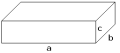(not drawn accurately)

a = 14 cm

b = 8.2 cm

c = 6.5 cm

(Just write the number)

Factorise

6x + 10y

The maximum daytime temperatures in ºC on 9 British summer days were as follows.

Work out the mean maximum temperature in ºC to 1 decimal place.

(Just write the number)

18,  17,  19,  18,  20,  15,  17,  18,  16

My garden consists of a rectangular lawn with a circular pond, as shown below.

Calculate the area of grass in m2 to 3 significant figures.

(Just write the number)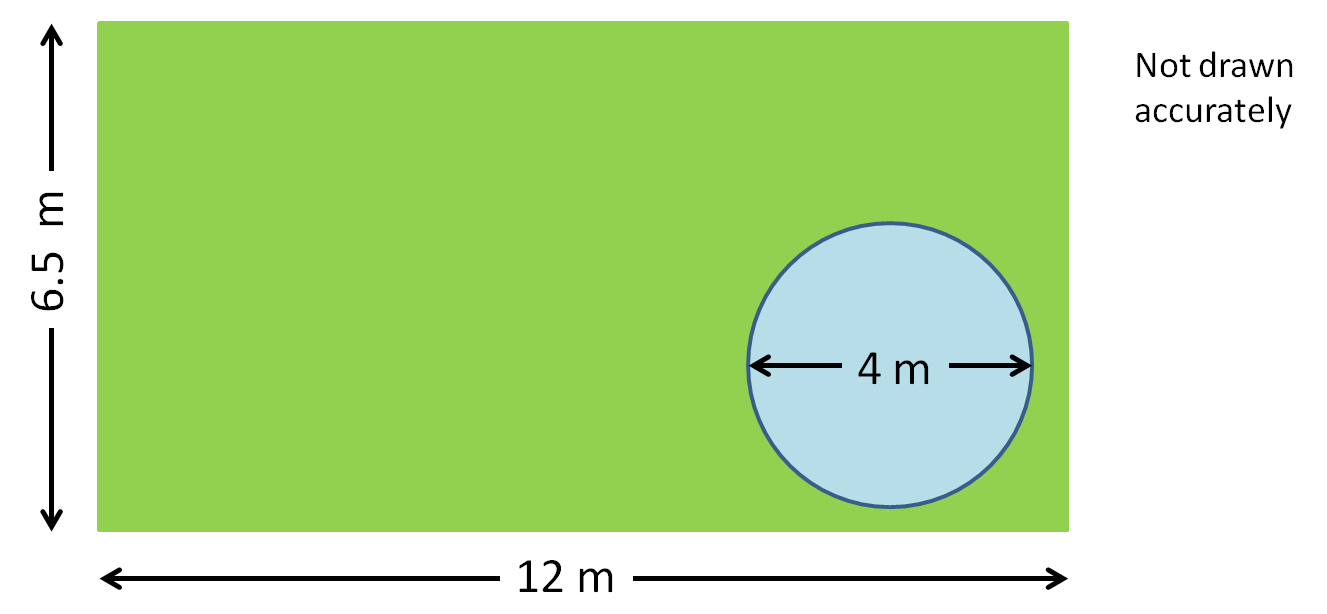• Question 1

Last year, the following number of people went to visit Leonardo Da Vinci's Last Supper:

Three hundred and two thousand, five hundred and thirty-seven.

(Write this number in figures)

302537
302,537
EDDIE SAYS
Three hundred and two thousand is written as 302000.
• Question 2

The diagram shows a protractor correctly positioned to measure the angle shown.

What is the size of the acute angle shown below.64°
EDDIE SAYS
Use the inner scale from 0° anticlockwise to 64° (i.e. 4 small markings past 60°)
• Question 3

Unlikely

Impossible

Certain

Likely

Even Chance

Match the words above to describe the likelihood of these five situations happening.

## Column B

There will be 100 days in Decembe...
Impossible
It will snow in Italy next August...
Unlikely
The next baby born in England wil...
Even Chance
I will get at least one head if I...
Likely
The sun will set tonight.
Certain
EDDIE SAYS
100 days in a month is not possible.
It is not likely to snow in Italy in August, but there is a slight chance perhaps in the mountains.
A boy and a girl are roughly equally likely to be born.
With such a large number of throws, it is likely that at least one will be a head.
The sun sets every evening.
• Question 4

Use a calculator to work out

the square root of 7.29

2.7
EDDIE SAYS
Use the Square Root (√) button.
• Question 5

Work out:

¾ of 128

96
EDDIE SAYS
On your calculator, work out 128 ÷ 4. This gives one quarter of 128.
Multiply by 3 to get three-quarters.
• Question 6

Pens cost £1.35 each.

Ann has £20 to spend on pens.  She buys the greatest number possible.

Work out the number of pens Ann buys.

14
EDDIE SAYS
20 ÷ 1.35 = 14.814814814....
She cannot buy 0.814814814... of a pen.
• Question 7

Solve:

2y - 5 = 12

y = 8½
EDDIE SAYS
2y - 5 = 12

Add 5 to both sides to get
2y = 17

Halve both sides to get
y = 8½
• Question 8

Calculate the area of the trapezium shown below in cm2.

(Just write the number)72
EDDIE SAYS
Area = ½(6 + 12)× 8 = ½ × 18 × 8 = 9 × 8 = 72 cm²
• Question 9

Write down the value of

142

196
EDDIE SAYS
This is 14 squared or 14 × 14
• Question 10

Write down the value of

43

64
EDDIE SAYS
This is 4 cubed or 4 × 4 × 4
• Question 11

From this list of number, match the answers.

15   17   19   21   23   25

## Column B

the smallest prime number
17
The largest prime number
23
A square number
25
A multiple of 7
21
EDDIE SAYS
The prime numbers are 17, 19 and 23.
25 is 5 squared.
21 is 3 × 7;
• Question 12

Round this number to 2 significant figures:

18742

19000
EDDIE SAYS
18742 is nearer to 19000 than to 18000.
The 1 and the 9 are the first two significant figures.
• Question 13

18.9 x 2.331

44.06
EDDIE SAYS
Using a calculator, the answer is 44.0559.
The third decimal place is 5, which is a high number, so the answer is 44.06
• Question 14

Ahmed goes on holiday to Italy and changes £750 into euros (€) before he goes.

The exchange rate is £1 = €1.12.

In Italy, he spends €480 euros and brings the remaining euros home.

He changes these euros back into pounds.

The exchange rate is now £1 = €1.20.

How much money in pounds does he get back?

(Just write the number)

300
EDDIE SAYS
£750 = 750 × 1.12 = €840
He spends €480 so has 840 - 480 = €360 left
€360 = 360 ÷ 1.20 = £300
• Question 15

Simplify

5a + 8b - 2a + b

3a+9b
EDDIE SAYS
Collect the a terms to get: 5a - 2a = 3a
Collect the b terms to get: 8b + b = 9b
• Question 16

Calculate the area of the following shape made of rectangular pieces.

(Just write the number)17.6
EDDIE SAYS
Divide the shape into a 3 by 3 square and a 4.3 by 2 rectangle.
Area = 3 × 3 + 2 × 4.3 = 9 + 8.6 = 17.6 m²
• Question 17

The Triangle ABC is isosceles.

Calculate the angle marked a°.74
EDDIE SAYS
∠ABC is opposite and equal to the angle marked as 32°
a° + a° = 180 - 32 = 148°
a° = 148 ÷ 2 = 74°
• Question 18

Calculate the length of the side BC in cm in the following triangle.

(Just write the number to 2 decimal places)9.49
EDDIE SAYS
Using Pythagoras' Theorem, BC² = 3² + 9² = 9 + 81 = 90

BC = √90 = 9.4868....
• Question 19

Here is a sign showing the prices for using a car park.

 CAR PARK £1.75 for the first hour £1.25 for each hour after

Jane pays £9.25 to park.

For how many hours does she park?

(Just write the number)

7
EDDIE SAYS
£9.25 - £1.75 = £7.50
£7.50 ÷ 1.25 = 6
• Question 20

Match the most suitable measurement to each item.

## Column B

Mass of a drawing pin
1g
Mass of an apple
100g
Height of a bus
4.4m
Height of a table
56cm
Height of Tower of Pisa
56m
• Question 21

Use trial and improvement to find a solution to the equation

x3 - 2x = 31

The first step is shown below.

Give the solution to 1 decimal place

x  x3 - 2x  Comment
3  33 - 2×3 = 27 - 6 = 21  too small

3.4
EDDIE SAYS
3.4³ - 2×3.4 = 32.504 too big
3.3³ - 2×3.3 = 29.337 too small
3.35³ - 2×3.35 = 30.895375 still too small
So answer lies between 3.35 and 3.4, which will give a solution of 3.4 to 1 decimal place.
• Question 22
 Find the volume of the following cuboid in cm3 to the nearest whole number.(not drawn accurately)

a = 14 cm

b = 8.2 cm

c = 6.5 cm

(Just write the number)

746
EDDIE SAYS
Volume = 14 × 8.2 × 6.5 = 746.2 cm³
• Question 23

Factorise

6x + 10y

2(3x+5y)
EDDIE SAYS
The HCF of 6 and 10 is 2, so 2 goes outside the brackets.
To find the inside terms, divide 6x and 10y by 2.
• Question 24

The maximum daytime temperatures in ºC on 9 British summer days were as follows.

Work out the mean maximum temperature in ºC to 1 decimal place.

(Just write the number)

18,  17,  19,  18,  20,  15,  17,  18,  16

17.6
EDDIE SAYS
The total is 158
158 ÷ 9 = 17.555555555... which rounds to 17.6
• Question 25

My garden consists of a rectangular lawn with a circular pond, as shown below.

Calculate the area of grass in m2 to 3 significant figures.

(Just write the number)65.4
EDDIE SAYS
Area of garden = 12 × 6.5 = 78 m²

Radius of pond = half of 4 m = 2 m
Area of pond = π × 2² = 12.566 m²

Area of grass = 78 - 12.566 = 65.434 ≅ 65.4 m²
---- OR ----

Sign up for a £1 trial so you can track and measure your child's progress on this activity.

### What is EdPlace?

We're your National Curriculum aligned online education content provider helping each child succeed in English, maths and science from year 1 to GCSE. With an EdPlace account you’ll be able to track and measure progress, helping each child achieve their best. We build confidence and attainment by personalising each child’s learning at a level that suits them.